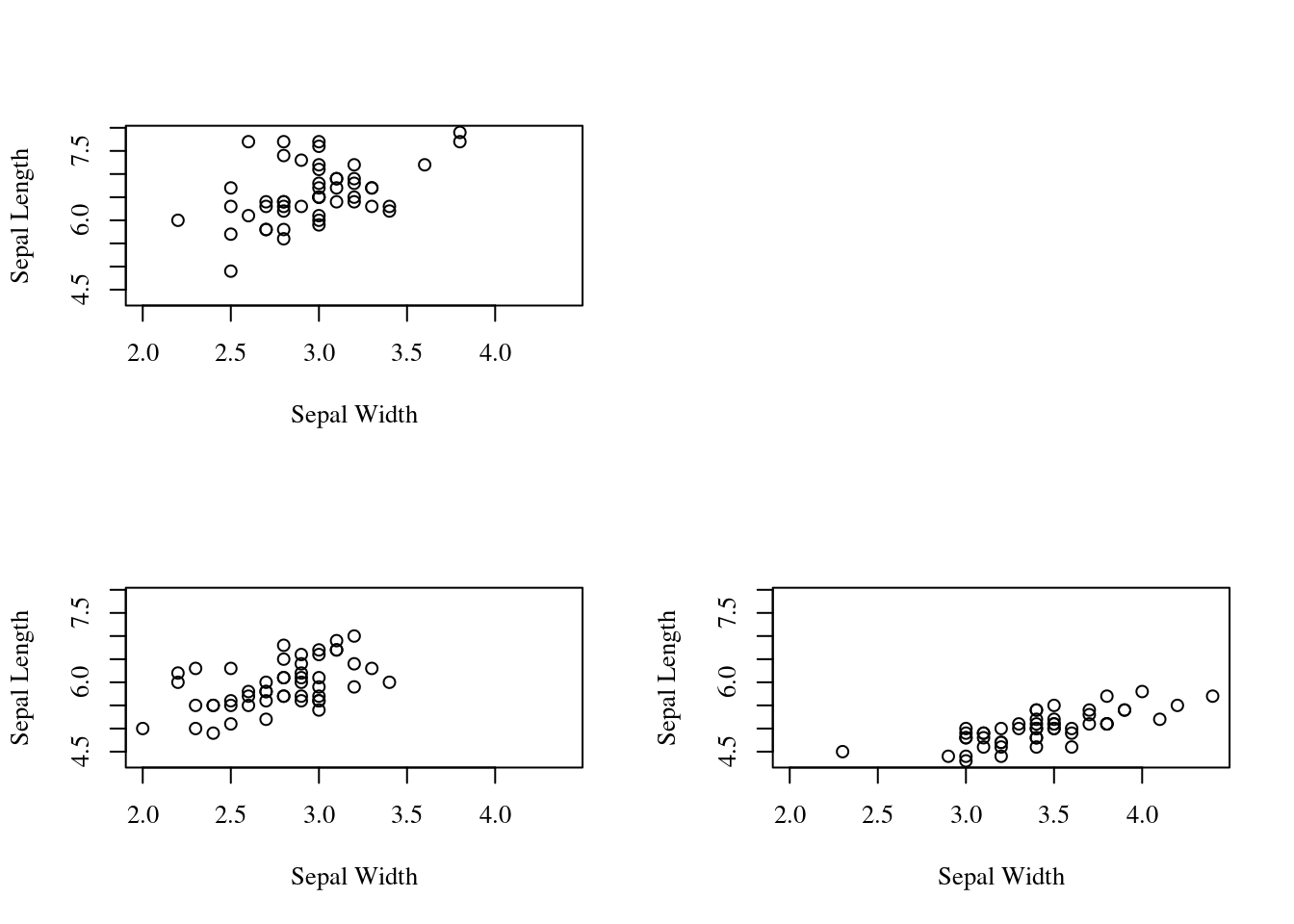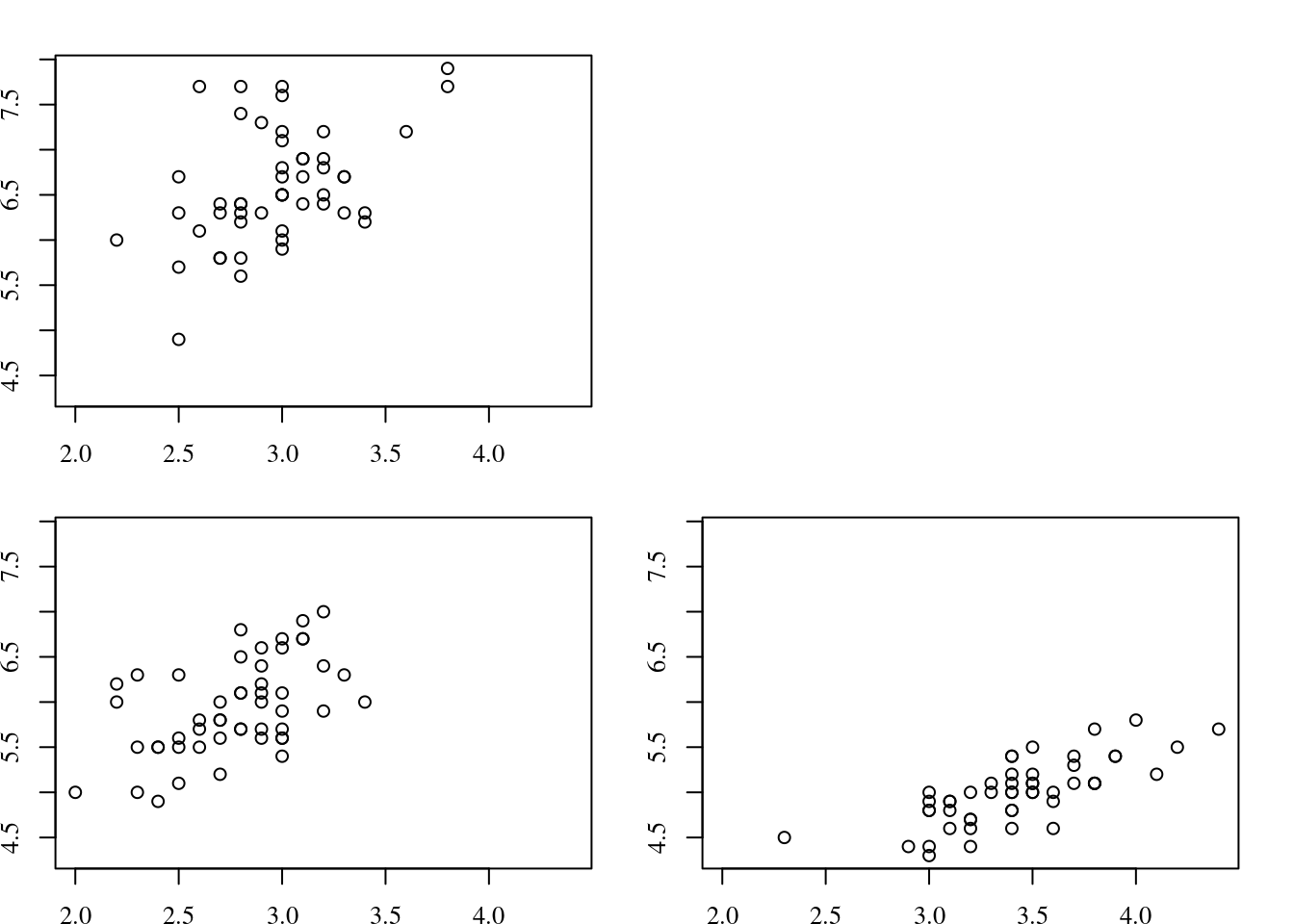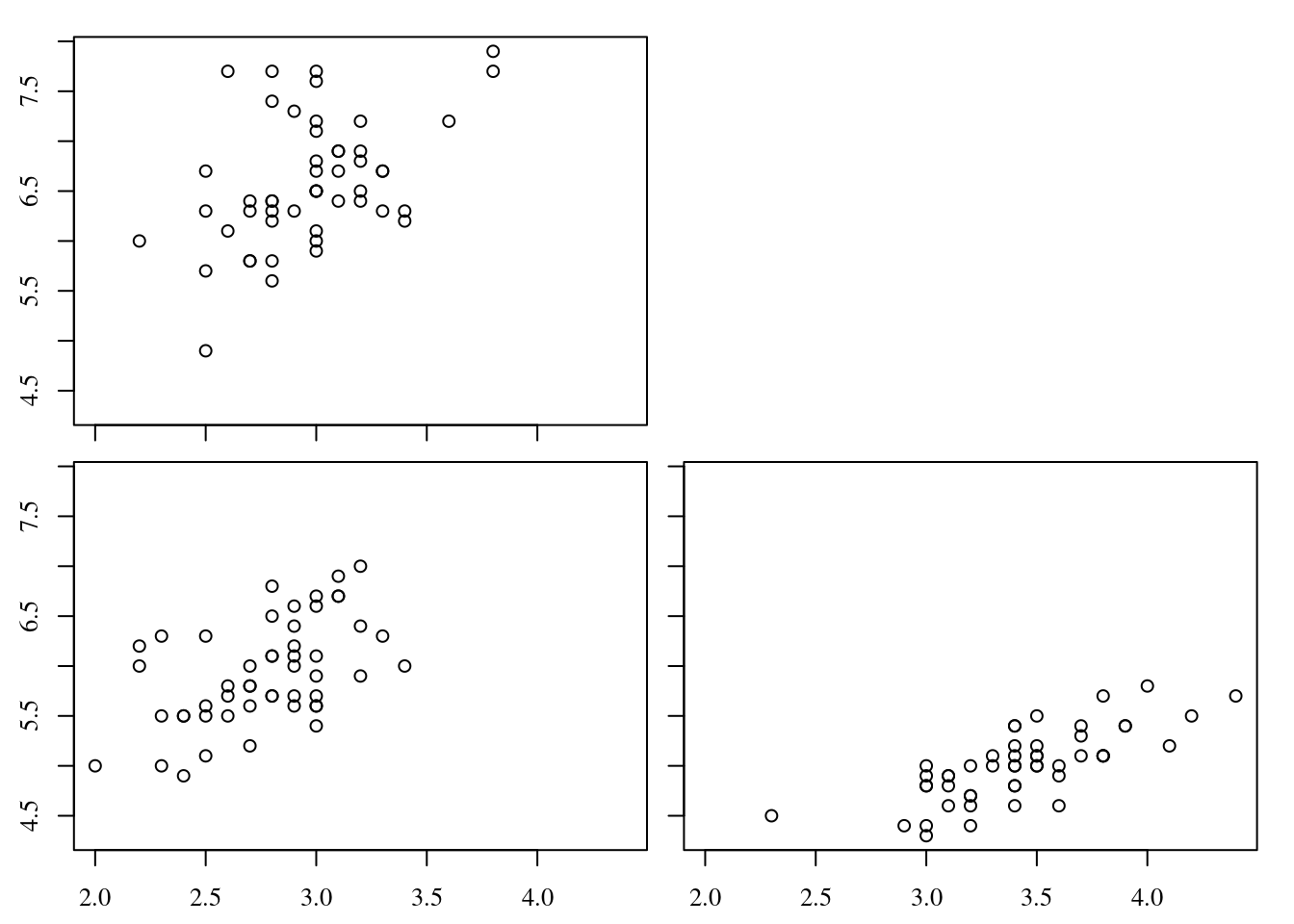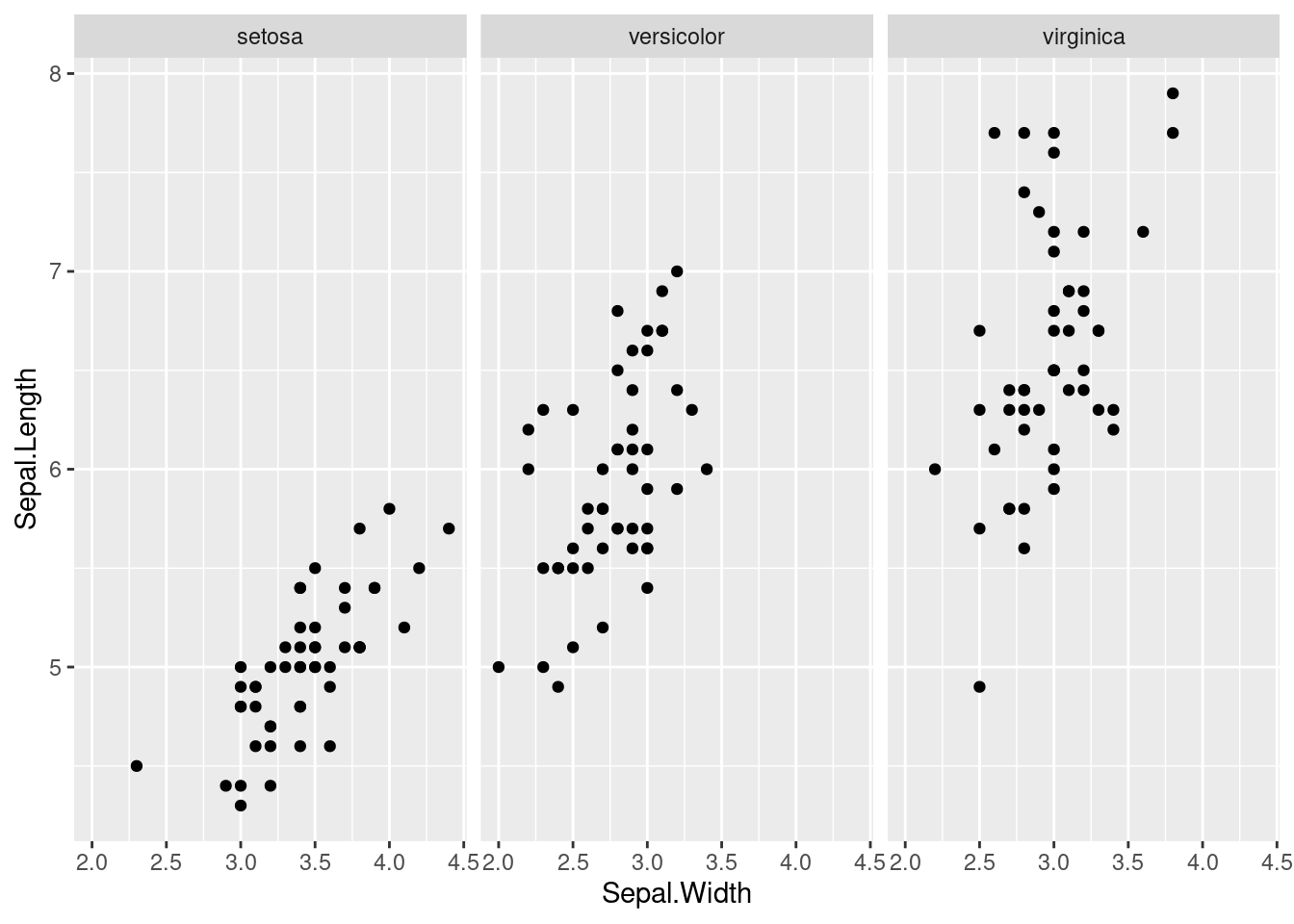# Spacing of multi-panel figures in R

In a previous post, I showed how to keep text and symbols at the same size across figures that have different numbers of panels. The figures in that post were ugly because they used the default panel spacing associated with the `mfrow` argument of the `par` function. Below I will walk through how to adjust the spacing of the panels when using `mfrow`.

For this example, we will use Edgar Anderson’s iris data, which is distributed with R. The data set includes flower measurements for 3 iris species. In this case, the data would be more effectively plotted in a single panel with different colors or symbols for each species, but with larger data sets the different colors/symbols can create a jumbled mess and multi-panel figures illustrate patterns in the data more clearly.

To plot all the data on the same scale, we need to extract the max and min values of the variables that we are plotting.

``````min.width = min(iris\$Sepal.Width)
min.length = min(iris\$Sepal.Length)
max.width = max(iris\$Sepal.Width)
max.length = max(iris\$Sepal.Length)``````

The next block of code plots 3 panels in a 2x2 arrangement1 with mostly default options. An empty panel is created by calling `plot.new`. The empty panel can be placed in any of the 4 positions, but it is redundant to use `plot.new` for the bottom right panel because `mfrow` fills the graphics device by row, moving left to right along the row.

``````par(mfrow=c(2,2), tcl=-0.5, family="serif")

# Top left panel
plot(Sepal.Length~Sepal.Width, data=iris, subset=(Species=="virginica"),
xlab="Sepal Width", ylab="Sepal Length", xlim=c(min.width,max.width),
ylim=c(min.length,max.length))

# Top right panel
plot.new()

# Bottom left panel
plot(Sepal.Length~Sepal.Width, data=iris, subset=(Species=="versicolor"),
xlab="Sepal Width", ylab="Sepal Length", xlim=c(min.width,max.width),
ylim=c(min.length,max.length))

# Bottom right panel
plot(Sepal.Length~Sepal.Width, data=iris, subset=(Species=="setosa"),
xlab="Sepal Width", ylab="Sepal Length", xlim=c(min.width,max.width),
ylim=c(min.length,max.length))``````Yikes! What a mess!

We can use the `mai` argument to the `par` function to specify the margin (in inches) of each panel in the figure. It takes a little trial-and-error to hit on margins that produce the desired spacing. We can start to reduce the redundancy in the figure by removing the labels for the x- and y-axes.

``````par(mfrow=c(2,2), tcl=-0.5, family="serif", mai=c(0.3,0.3,0.3,0.3))

# Top left panel
plot(Sepal.Length~Sepal.Width, data=iris, subset=(Species=="virginica"),
xlab=" ", ylab=" ", xlim=c(min.width,max.width), ylim=c(min.length,max.length))

# Top right panel
plot.new()

# Bottom left panel
plot(Sepal.Length~Sepal.Width, data=iris, subset=(Species=="versicolor"),
xlab=" ", ylab=" ", xlim=c(min.width,max.width), ylim=c(min.length,max.length))

# Bottom right panel
plot(Sepal.Length~Sepal.Width, data=iris, subset=(Species=="setosa"),
xlab=" ", ylab=" ", xlim=c(min.width,max.width), ylim=c(min.length,max.length))``````Not bad, but we still have redundancy by including numbers on the x-axis scale in the top left panel and the y-axis scale in the bottom right panel. When we drop the numbers from those panels, it will create more white space. But we want to use as much white space as possible for plotting. Instead of including only a single call to the `par` function to change the `mai` argument, we will call `par` before plotting each panel to customize the size of the margin on each side of the plot for each panel. The `mai` argument specifies the margin by sides, i.e., `c(bottom, left, top, right)`.

In the top left panel, we want a smaller bottom margin because we are going to drop the numbers from the x-axis scale. In the bottom left panel, we want larger margins on the bottom and left because we need to include the axis scales on those sides. Again, you simply use trial-and-error to get the margins how you want them, but there is one little catch. If you want all of your panels to have plots that fill the same area, then the bottom+top and left+right margins need to be the same in all panels. In our example, the sum is 0.4 for both, but bottom+top does not need to equal left+right.

``````par(mfrow=c(2,2), tcl=-0.5, family="serif")

# Top left panel
par(mai=c(0.2,0.4,0.2,0))
plot(Sepal.Length~Sepal.Width, data=iris, subset=(Species=="virginica"),
xlab=" ", ylab=" ", xlim=c(min.width,max.width), ylim=c(min.length,max.length), xaxt="n")
axis(1, labels=FALSE)

# Top right panel
plot.new()

# Bottom left panel
par(mai=c(0.4,0.4,0,0))
plot(Sepal.Length~Sepal.Width, data=iris, subset=(Species=="versicolor"), xlab=" ", ylab=" ",
xlim=c(min.width,max.width), ylim=c(min.length,max.length))

# Bottom right panel
par(mai=c(0.4,0.2,0,0.2))
plot(Sepal.Length~Sepal.Width, data=iris, subset=(Species=="setosa"), xlab=" ", ylab=" ",
xlim=c(min.width,max.width), ylim=c(min.length,max.length), yaxt="n")
axis(2, labels=FALSE)``````The panels are now well placed within the figure. We just need to add a little extra space around the outer margin to place our axis labels. The `omi` argument to the `par` function specifies the outer margins (in inches) with the sides listed in the same order as for `mai`. The `mtext` function allows you to place text in the outer margin. I have also labeled each panel by species, which required some small adjustments to the y-axis scale.

``````par(mfrow=c(2,2), tcl=-0.5, family="serif", omi=c(0.2,0.2,0,0))

# Top left panel
par(mai=c(0.2,0.4,0.2,0))
plot(Sepal.Length~Sepal.Width, data=iris, subset=(Species=="virginica"), xlab=" ", ylab=" ",
xlim=c(min.width,max.width), ylim=c(min.length,max.length+0.15), xaxt="n", bty="n")
axis(1, labels=F)
text((max.width-min.width)/2 + min.width, max.length+0.15, expression(italic("Iris virginica")))

# Top right panel
plot.new()

# Bottom left panel
par(mai=c(0.4,0.4,0,0))
plot(Sepal.Length~Sepal.Width, data=iris, subset=(Species=="versicolor"), xlab=" ", ylab=" ", xlim=c(min.width,max.width), ylim=c(min.length,max.length+0.15), bty="n")
text((max.width-min.width)/2 + min.width, max.length+0.15, expression(italic("Iris versicolor")))

# Bottom right panel
par(mai=c(0.4,0.2,0,0.2))
plot(Sepal.Length~Sepal.Width, data=iris, subset=(Species=="setosa"), xlab=" ", ylab=" ",
xlim=c(min.width,max.width), ylim=c(min.length,max.length+0.15), yaxt="n", bty="n")
axis(2, labels=F)
text((max.width-min.width)/2 + min.width, max.length+0.15, expression(italic("Iris setosa")))

mtext("Sepal Width", side=1, outer=T, at=0.5)
mtext("Sepal Length", side=2, outer=T, at=0.5)``````We now have a figure that is several steps closer to being publication quality. The main adjustment left to make is customizing the scales of the axes. In another post, I demonstrate how to customize the axes scales, which is particularly useful when presenting transformed data on the original scale.

Lastly, I want to point out how useful the `lattice` package is for quickly generating multi-panel figures with grouped data. As in the R base package, the default is not publication quality, but it requires a lot less code to generate a multi-panel figure that is at least easily readable.

``````library(lattice)
xyplot(Sepal.Length~Sepal.Width|Species,data=iris)``````Similarly, `ggplot2` allows for quickly creating multi-panel figures.

``````library(ggplot2)
ggplot(iris, aes(x = Sepal.Width, y = Sepal.Length)) +
geom_point() +
facet_wrap(~Species)``````1. The optimal way to plot this data in a multi-panel figure is a 3x1 arrangement, but using the 2x2 arrangement provides a better illustration of how `mfrow` works.↩︎